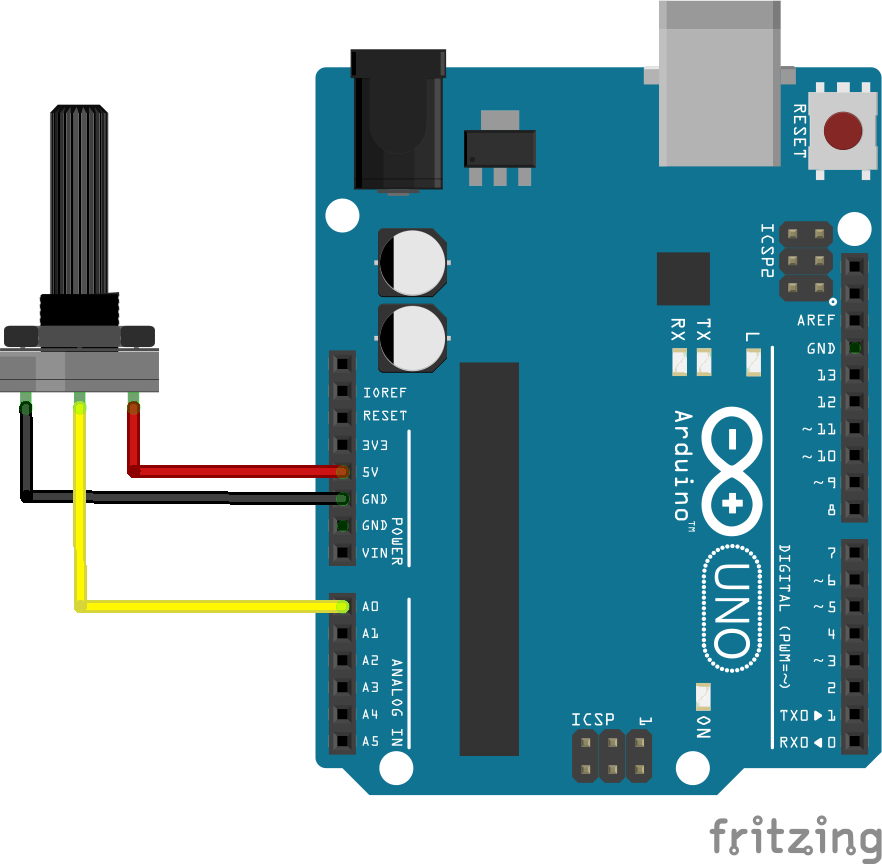# What is the Serial Communication?

Serial communication is the process of sending data one bit at a time, sequentially, over a communication channel or computer bus. Simply put, serial communication is the communication between two or more computers with binary data.

In this tutorial, we will use serial communication protocol to send data to Processing from Arduino. The Processing sketch will be controlled by the physical component, the potentiometer, which can be replaced with other sensors, button and etc.

# Wiring

Wiring up buttons and switches is simple:

1. Left pin to 5V
2. Right pin to GND
3. middle pin to A0# Arduino Code

This example sends the potentiometer value measured from Arduino to Processing via the serial port, you can read the data from the serial monitor.

``````#define potPin A0
int value;

void setup() {
Serial.begin(9600); //intailise Serial communication with 9600 baud rate
pinMode( potPin, INPUT );
}

void loop() {
Serial.println(value); //read the sensor and send the value to the Serial
delay(100); //little delay to prevent Arduino going crazy
}
``````

# Processing Code

This example used the data received from Arduino to control the degrees of rotation of a rectangle in Processing.

``````import processing.serial.*;
Serial myPort;
String val;

int datanum = 0; //number of data receiving from Arduino

int value1;
//int value2; //multiple data from arduino if needed

void setup() {
size(800, 800);

printArray(Serial.list()); //show all ports
String portName = Serial.list();//choose the correct port
myPort = new Serial(this, portName, 9600);
myPort.bufferUntil('\n');

}

void draw() {
//map() is a important function to use here,
//it convert the raw data range from Arduino to the ideal range to use in Processing
//map(a, b, c, d, e) has 5 Parameters
//map(theVariableYouWantToMap, min.ValueOfRawData, max.ValueOfRawData, min.ValueOfIdealRange, max.ValueOfIdealRange)

//in this case the min. value from the pot is 0 and max. value is 1023.
//and I want to map the background colour from black to white, 0(black) - 255(white)
float BW = map(value1,0, 1023, 0, 255 ); //create a variable to contain the converted value
background(BW);

}

void serialEvent( Serial myPort){
if (val != null)
{
val = trim(val);
int[] vals = int(splitTokens(val, ","));

if(vals.length >= datanum){
value1 = vals;

//multiple data from arduino if needed
//value2 = vals ;

print(value1);
}
}
}
``````